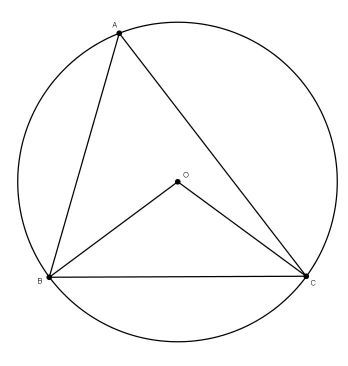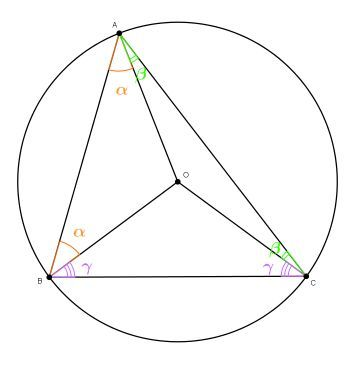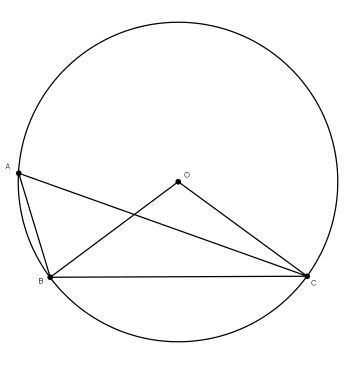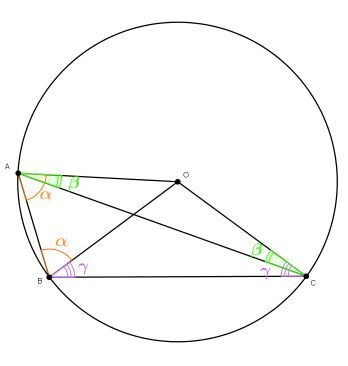# Voice typo?

• At timestamp 1:10 it seemed like Prof. Loh just repeated what he said. Also when or where can I find proof of why inscribed angles= 1/2 the angles they inscribe? I forgot sorry.

The formal wording of this theorem goes like this:

Inscribed Angle Theorem:
Any angle that is inscribed in a circle is half of the central angle that subtends the same arc on the circle.

Proof:
Let our inscribed angle be $$\angle BAC$$ and the central angle $$\angle BOC.$$
There are two cases for the position of the inscribed angle relative to the central angle.

Case 1:Let's draw one more radius, $$\overline{OA}.$$ Now we have three isosceles triangles: $$\bigtriangleup AOB,$$ $$\bigtriangleup AOC$$ and $$\bigtriangleup BOC.$$ So the the angles at the base are equal. Let $$\alpha = \angle BAO=\angle ABO,$$ $$\beta = \angle CAO=\angle ACO$$ and $$\gamma= \angle BCO=\angle CBO.$$ So the $$\angle BAC = \alpha+\beta.$$Let's now find the measure of the angle $$\angle BOC:$$
The sum of the angles in any triangle is equal to $$180^{\circ},$$ so $$\angle BOC=180^{\circ}-2\gamma$$ and also $$\angle BAC+\angle ABC+\angle ACB=180^{\circ}.$$
On the other hand, $$\text{sum of the angles in }\triangle ABC=2\alpha+2\beta+2\gamma=180^{\circ},$$ hence $$2\alpha+2\beta = 180^{\circ}-2\gamma=\angle BOC.$$ And we got what we wanted: $$\angle BOC=2\alpha+2\beta=2(\alpha+\beta)=2\angle BAC.$$
So we are done!

Case 2:
Now, if you want, you can try to prove this case yourself, using the ideas from the proof of the first caseLet's draw one more radius, $$\overline{OA}.$$ Now we have three isosceles triangles: $$\bigtriangleup AOB,$$ $$\bigtriangleup AOC$$ and $$\bigtriangleup BOC.$$ So the angles at the base are equal. Let $$\alpha = \angle BAO=\angle ABO,$$ $$\beta = \angle CAO=\angle ACO$$ and $$\gamma= \angle BCO=\angle CBO.$$ So the $$\angle BAC = \alpha-\beta.$$Let's now find the measure of the angle $$\angle BOC:$$
The sum of the angles in any triangle is equal to $$180^{\circ},$$ so $$\angle BOC=180^{\circ}-2\gamma$$ and also $$\angle BAC+\angle ABC+\angle ACB=180^{\circ}.$$
On the other hand, $$\text{sum of the angles in }\triangle ABC=(\alpha-\beta)+(\alpha+\gamma)+(\gamma-\beta) = 2\alpha-2\beta+2\gamma=180^{\circ},$$ hence $$2\alpha-2\beta = 180^{\circ}-2\gamma=\angle BOC.$$ And we got what we wanted: $$\angle BOC=2\alpha-2\beta=2(\alpha-\beta)=2\angle BAC.$$
So we are done again, using the same algorithm!

• @nastya $$\bigtriangleup ACO$$